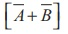Home | | Physics 12th Std | Verification of De MorganŌĆÖs Theorems

# Verification of De MorganŌĆÖs Theorems

To verify De MorganŌĆÖs first and second theorems.

VERIFICATION OF DE MORGANŌĆÖS THEOREMS

## AIM:

To verify De MorganŌĆÖs first and second theorems.

## COMPONENTS REQUIRED:

Power Supply (0 ŌĆō 5V), IC 7400, 7408, 7432, 7404, and 7402, Digital IC trainer kit, connecting wires.

## FORMULA## CIRCUIT DIAGRAM:

De MorganŌĆÖs first theorem, De MorganŌĆÖs second theorem## i) Verification of De MorganŌĆÖs first theorem

┬Ę        The connections are made for LHSof the theorem as shown in the circuit diagram using appropriate ICs.

┬Ę        The output is noted and tabulated for all combinations of logical inputs of the truth table.

┬Ę        The same procedure is repeated for RHSof the theorem.

┬Ę        From the truth table, it can be shown that## ii) Verification of De MorganŌĆÖs second theorem

┬Ę        The connections are made for LHSof the theorem as shown in the circuit diagram using appropriate ICs.

┬Ę        The output is noted and tabulated for all combinations of logical inputs of the truth table.

┬Ę        The same procedure is repeated for RHSof the theorem.

┬Ę        From the truth table, it can be shown that## OBSERVATION

De-MorganŌĆÖs first theorem, De-MorganŌĆÖs second theorem### RESULT

De MorganŌĆÖs first and second theorems are verified.

### Note

The pin diagram for IC 7408, IC 7432 and IC 7404 can be taken from previous experiment

Precautions

VCC and ground pins must not be interchanged while making connections. Otherwise the chip will be damaged.

For the ICs used, 5V is applied to the pin 14 while the pin 7 is connected to the ground.

Tags : Physics Practical Experiment , 12th Physics : Practical
Study Material, Lecturing Notes, Assignment, Reference, Wiki description explanation, brief detail
12th Physics : Practical : Verification of De MorganŌĆÖs Theorems | Physics Practical Experiment